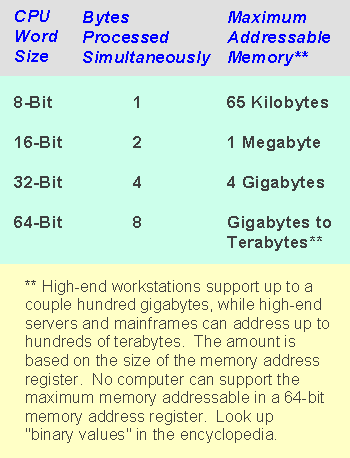# 8-bit computing

(redirected from 8 bits)

## 8-bit computing

CPUs that process 8 bits as a single unit. The first personal computers in the late 1970s, such as the Apple II, Radio Shack TRS-80 and various Z80-based CP/M computers, all used 8-bit CPUs. Personal computers migrated to 16 bits when IBM selected the Intel 8088 CPU for the PC and later to 32 bits with the Intel 386. Today, low-cost 8-bit processors are made by the billions and embedded in myriad devices (see 8-bit MCU). See 8088, 386, 16-bit computing and bit specifications.Mentioned in ?
References in periodicals archive ?
The integer notation is defined here as follows: [N.sub.D] is the digit expression (either in 8 bits or 16 bits), [N.sub.B] is the binary expression of [N.sub.D], and [N.sub.U] is the unary expression.
First part is the cache Tag memory (also named Tag RAM), the size of this memory is 8K x 8 bits and the second part is the cache RAM memory (also named Data RAM).
Both also support 12 bits per RGBA component (versus 8 bits found in other workstations), thereby capable of displaying 68 billion colors (versus the typical workstation, which can display 16 million colors).
Thus the bandwidth is: 25MHz strobe, multiplied by 2 for double data rate clocking, then multiplied by 16 bits per edge, then divided by 8 bits per byte, which equals 100MB/sec.
The AES validation test suite tests the Modes of Operation ECB, CBC, OFB, CFB (1 bit, 8 bit, and 128 bit), and CTR.

Site: Follow: Share:
Open / Close